# Precalculus : Graphing Functions

## Example Questions

1 2 5 6 7 8 9 10 11 13 Next →

### Example Question #6 : Trigonometric Graphs (All Six)

Which of the following functions is represented by this graph?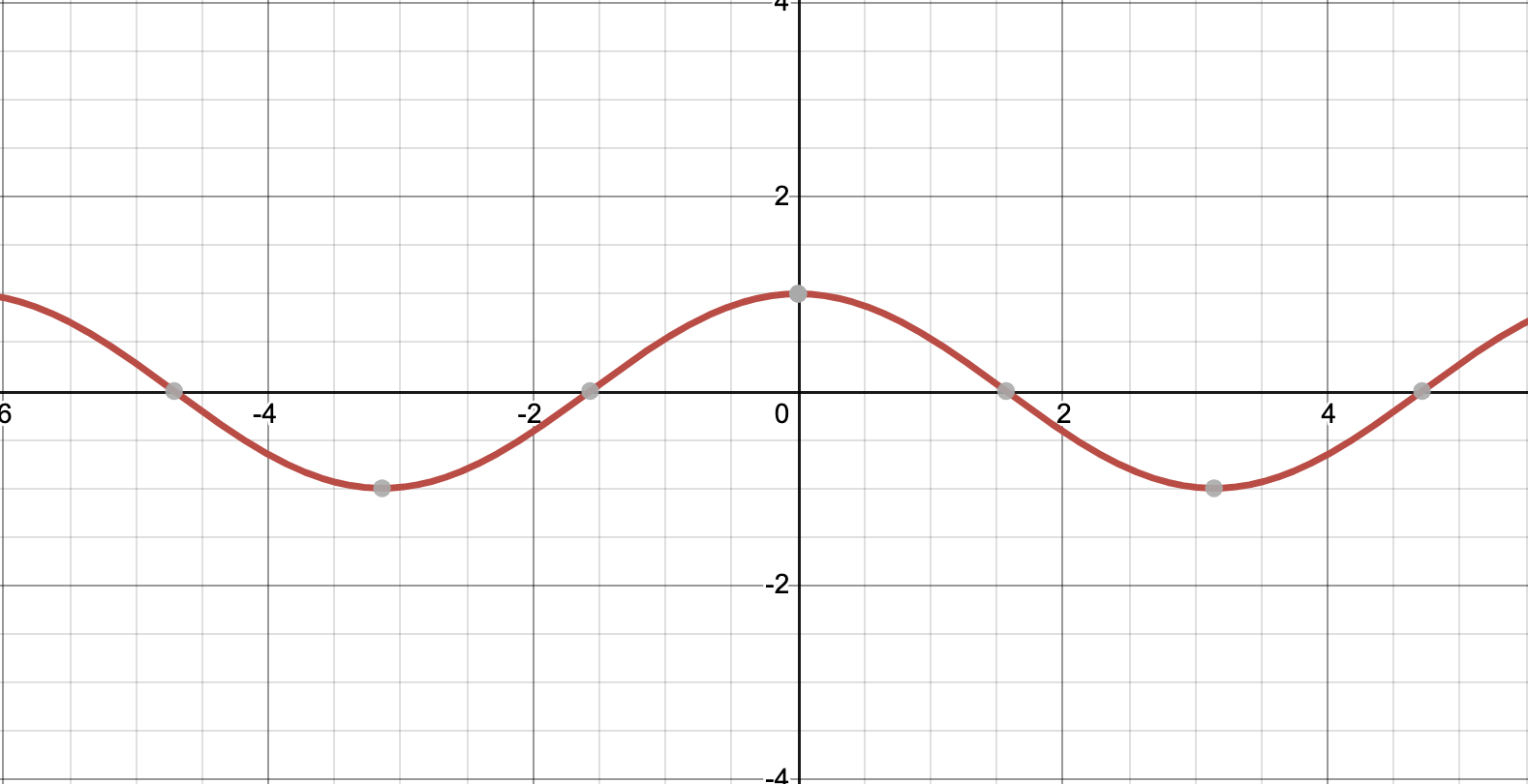Possible Answers:

y = cos(x)

y = tan(x)

y = sin(x)

y = csc(x)

y = sec(x)

Correct answer:

y = cos(x)

Explanation:

This graph is the graph of y = cos x. The domain of this function is all real numbers. The range of this function is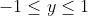. The period of this function is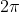.

### Example Question #7 : Trigonometric Graphs (All Six)

Which of the following functions is represented by this graph?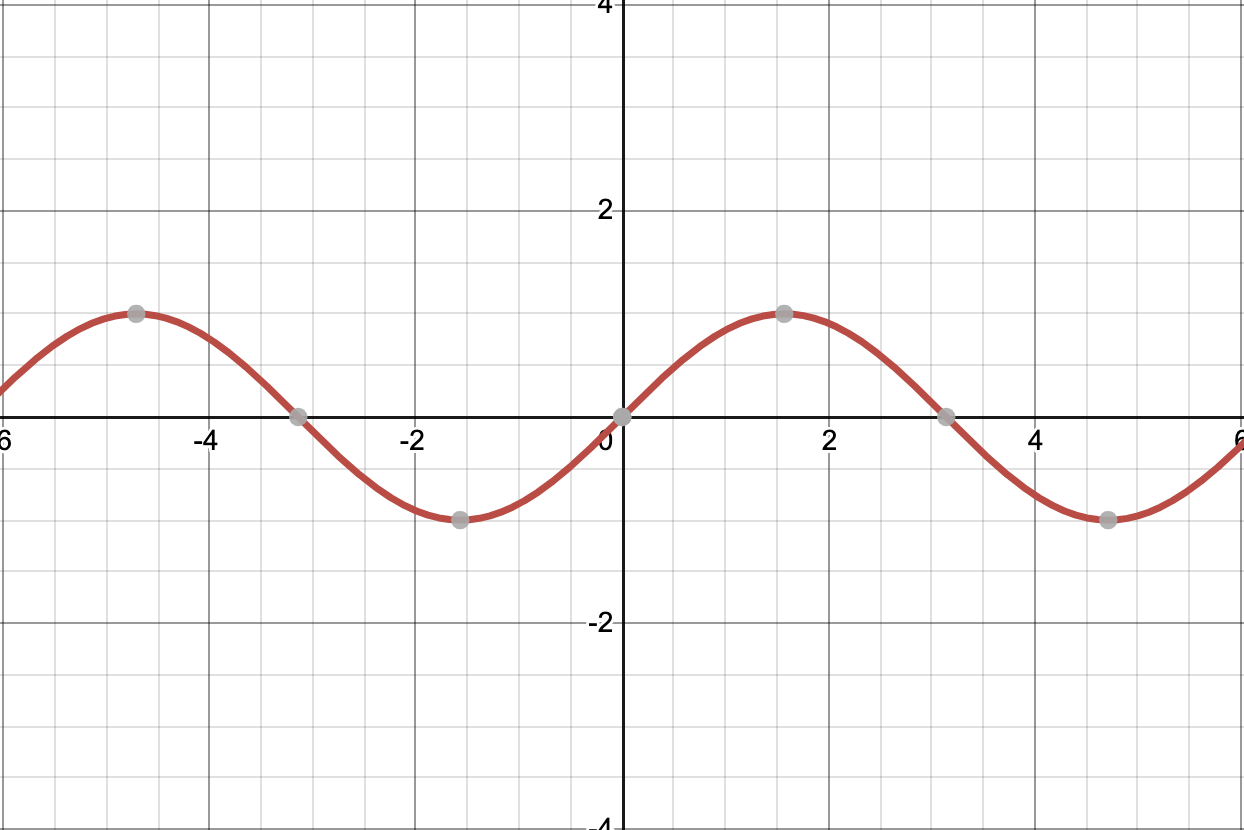Possible Answers:

y = tan x

y = csc x

y = sec x

y = cos x

y = sin x

Correct answer:

y = sin x

Explanation:

This graph is the graph of y = sin x. The domain of this function is all real numbers. The range of this function is. The period of this function is.

### Example Question #8 : Trigonometric Graphs (All Six)

True or false: If you translate a secant function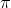units to the left along the x-axis, you will have a cosecant curve.

Possible Answers:

True

False

Correct answer:

False

Explanation:

This is false. While the graphs of secant and cosecant functions are related, in order to turn a secant function into a cosecant function, you'd need to translate the original graph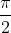units to the right to obtain a cosecant graph.

### Example Question #9 : Trigonometric Graphs (All Six)

Where does the tangent function intercept the x-axis?

Possible Answers: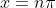No solution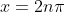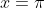x= all real numbers

Correct answer:Explanation:

Because the tangent function is periodic, it intercepts the x-axis in infinitely many places. We can see several of these in the graph below: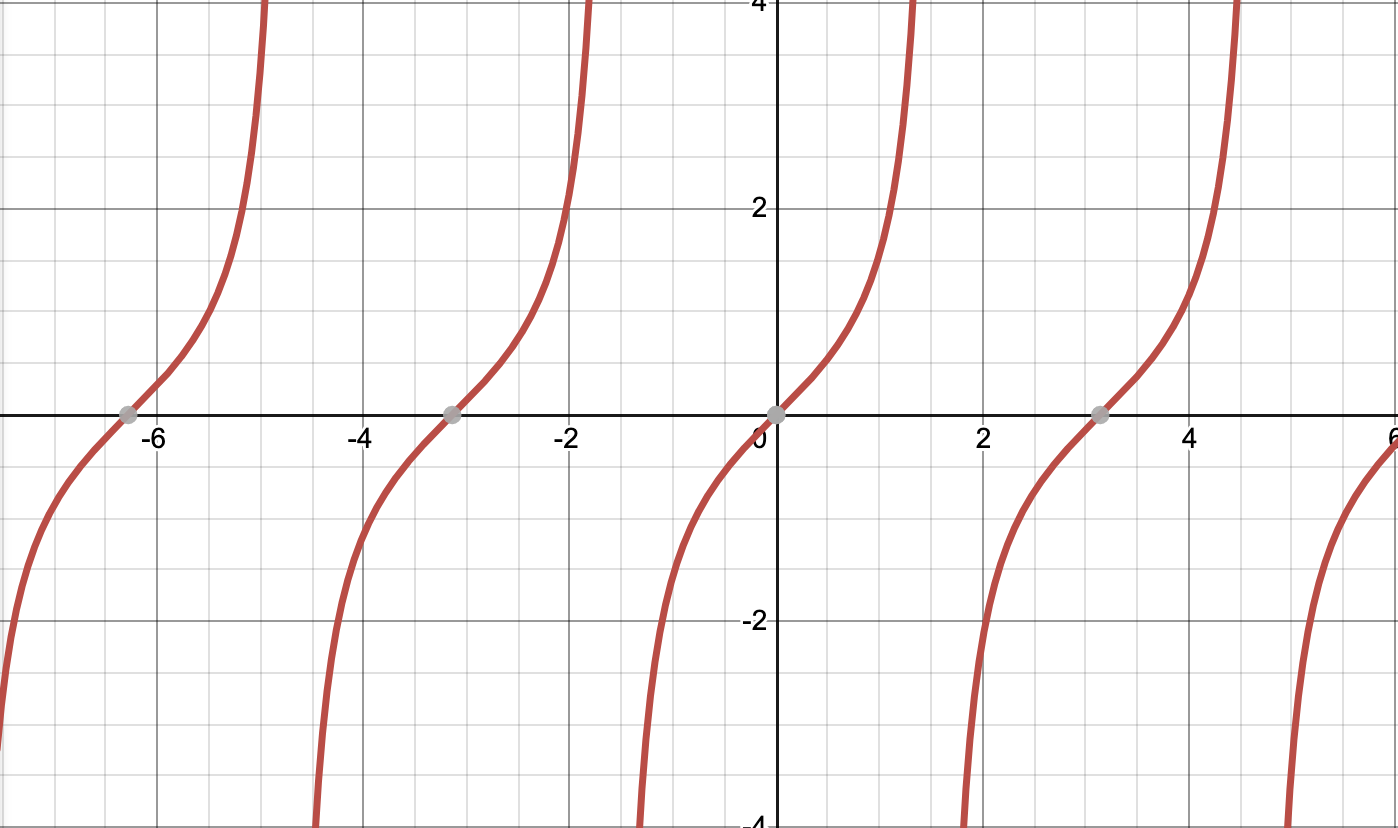In the photo, we can see that the function is intercepting the x-axis at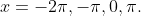Generalizing this, we can say that the tangent function intercepts the x-axis forfor all values of n such that n is an integer.

### Example Question #10 : Trigonometric Graphs (All Six)

True or false: If you translate a sine curve 90o to the left along the x-axis, you will have a cosine curve.

Possible Answers:

False

True

Correct answer:

True

Explanation:

This is true! Notice the similarity of the shape between the graphs, but that they intercept the x-axis at different spots, and their peaks and valleys are at different spots.

y=sin(x), passes through the point (0,0)y=cos(x), passes through the point (0,1)1 2 5 6 7 8 9 10 11 13 Next →

### All Precalculus Resources